Skip to main content

# CRLBs for WSNs localization in NLOS environment

## Abstract

Determination of Cramer-Rao lower bound (CRLB) as an optimality criterion for the problem of localization in wireless sensor networks (WSNs) is a very important issue. Currently, CRLBs have been derived for line-of-sight (LOS) situation in WSNs. However, one of major problems for accurate localization in WSNs is non-line-of-sight (NLOS) propagation. This article proposes two CRLBs for WSNs localization in NLOS environment. The proposed CRLBs consider both the cases that positions of reference devices (RDs) are perfectly or imperfectly known. Since non-parametric kernel method is used to build probability density function of NLOS errors, the proposed CRLBs are suitable for various distributions of NLOS errors. Moreover, the proposed CRLBs provide a unified presentation for both LOS and NLOS environments. Theoretical analysis also proves that the proposed CRLB for NLOS situation becomes the CRLB for LOS situation when NLOS errors go to 0, which gives a robust check for the proposed CRLB.

## Introduction

Wireless sensor networks (WSNs) have been widely used for monitoring and control in military, environmental, health, and commercial systems . A WSN usually consists of tens or hundreds of wirelessly connected sensors. Sensor positioning becomes an important issue. Since global positioning system (GPS) is currently a costly solution, only a small percentage of sensors are equipped with GPS receivers called reference devices (RDs), whereas the other sensors are blindfolded devices (BDs).

Several methods have been proposed to estimate the positions of sensors in WSNs. Multi-dimensional scaling (MDS) methods have been successfully applied to the problem of sensor localization in WSNs . These classic MDS approaches based on principal component analysis may not scale well with network size as its complexity is cubic in the number of sensors. A popular alternative to principal component analysis is the use of gradient descent or other numerical optimizations . The weighted version of MDS in  utilized the weighted cost function to improve positioning accuracy. The semi-definite programming algorithm in  was devised for WSNs localization in the presence of the uncertainties in the positions of RDs. Besides the above localization algorithms, some studies have been reported on performance analyses for WSNs localization . The authors in  derived the Cramer-Rao lower bounds (CRLBs) for the received-signal-strength and time-of-arrival (TOA) location technologies in WSNs. A more practical CRLB based on the distance-dependent variance model for range estimation noise was proposed in . In , the clock biases were considered in the CRLB for distributed positioning in sensor network. The authors in  proposed the CRLB for RD-free localization and derived the lower and upper bounds on the CRLB. Furthermore, the CRLBs considering the uncertainties in the positions of RDs were presented in [12, 15].

It should be noted that the above studies  are based on line-of-sight (LOS) assumption which may lead to severe degradations since non-line-of-sight (NLOS) propagation is a main problem for accurate localization in actual WSNs system. In the cellular location system (CLS) and local positioning system (LPS), some localization methods and performance analyses for NLOS environment have been addressed in the literature . The CRLB based on exponential distribution model in  cannot be used for other distributions of NLOS errors. The CRLB in  was derived for NLOS environment based on a single reflection model, and may not be accurate for a practical environment where most signals arrive at the receiver after multi-reflections. The CRLB with or without NLOS statistics was derived for NLOS situation . For the case without NLOS statistics, the authors  computed the CRLB in a mixed NLOS/LOS environment and proved that the CRLB for a mixed NLOS/LOS environment depends only on LOS signals. However, the CRLB without NLOS statistics  is not suitable for the situation where measurements from all base stations are corrupted by NLOS errors. For the case with NLOS statistics, the authors  only provided a definition of CRLB. The detail procedure and formulas for determining the CRLB under various distributions of NLOS errors in a practical environment were not considered in . Furthermore, multipath effects were considered in the CRLB for CLS and LPS [23, 24] and the LOS/NLOS unification was discussed in [23, 25]. In this article, two CRLBs compatible for various distributions of NLOS errors for WSNs localization in NLOS environment are proposed. Compared with the previous performance studies for NLOS situation , three main contributions of this article are listed as follows:

1. 1.

All the existing CRLBs for NLOS situation were devised only for CLS and LPS. For a WSNs location system, the problem of sensor localization becomes more complex since the range measurements among all sensors are used rather than limited measurements between a BD and RDs for a CLS and LPS. The proposed CRLBs considering all of range measurements among sensors can be used for not only WSNs location system but also CLS and LPS.

2. 2.

The proposed CRLBs based on non-parametric kernel method can be applicable for different cases including various distributions of NLOS errors, single or multi-reflections model.

3. 3.

Some characteristics of the CRLBs for WSNs localization are derived in this article. The proposed CRLB provides a unified CRLB presentation for both LOS and NLOS environments as shown in . This means that the previous research results for the CRLB of LOS environment can also be used for the CRLB of NLOS environment. For example, the poisoning accuracy increases as the more devices are used for sensor localization. Theoretical analysis shows that the CRLB for NLOS environment becomes the CRLB for LOS environment in the case that NLOS errors go to 0, which gives a robust check for the proposed CRLB.

This article is organized as follows. Signal model and some basic notations are presented in next section. Followed by kernel method is used to estimate the probability density function (PDF) of NLOS errors. Based on the estimated PDF, CRLBs for NLOS environment are derived. Next section proves a characteristic of CRLBs. Then, the CRLBs are evaluated by simulations. Finally, conclusions of this article are given.

### System model

Consider a TOA-based WSNs location system with n + m devices. Devices 1...n are BDs with the unknown coordinates, whereas devices n + 1...n + m are RDs with the known coordinates. Assume that (x i , y i ) is the position of the i th device. The vector of unknown parameters is: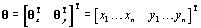(1)

As , devices may make incomplete observations due to the limited link capacity. Let H(i) = {j: device j makes pair-wise observations with device i}. Note that a device cannot measure range with itself, so that i H(i). It is also obvious that if j H(i) then i H(j).

The range measurement r ij between the i th and j th devices can be modeled as:(2)

where d ij is the true distance between the i th and j th devices, n ij represents the Gaussian noise with zero mean and variance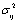, and b ij is NLOS error which may have different statistical distributions in practical channel environments. The residual noise is given by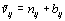. It should be noted that Gaussian noise n ij and NLOS error b ij are independent. This assumption has been widely used in the literature .

The CRLB can be used to determine the physical impossibility of the variance of an unbiased estimator being less than the bound . Let r be a vector of range measurements: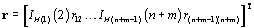(3)

where IH(k)(l) is an indicator function: 1 if l H (k) or 0 otherwise. The CRLB matrix is defined as the inverse of the Fisher information matrix (FIM) J: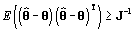(4)

where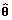is an estimate of θ.

The FIM is determined by :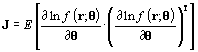(5)

The log of the joint conditional PDF is: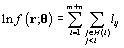(6)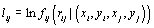(7)

Substituting (6) into (5), the FIM can be rewritten as :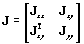(8)

where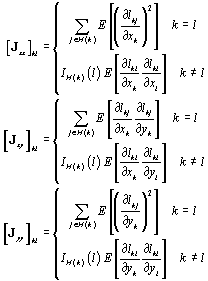(9)

### CRLBs in NLOS environment

#### Modeling range measurements

The derivation of CRLB is based on the PDF of NLOS errors. There are two methods for evaluating f(bij). The parametric method can only be used for specific noise distributions such as Gaussian, exponential, uniform, and delta distributions. The non-parametric method can be used for all noise distributions including the PDF without explicit expression.

The second method is developed to derive the CRLB for NLOS environment in this article. The basic procedure of non-parametric estimation is to create an approximation of the PDF from a given set of survey measurements. Assume that a survey set of NLOS errors {Sbij1...Sb ijP } with the size P is available for a propagation channel between the i th and j th devices. The estimated PDF of b ij can be obtained using non-parametric kernel method :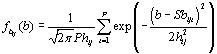(10)

where exp (·) is a Gaussian kernel function, the smoothing constant h ij is the width of the kernel function which can be determined by using the method in . Simulation results show that Equation 10 can perfectly estimate the PDF of NLOS errors from the survey set. Many non-parametric estimators such as histogram method, orthogonal series, and other kernel methods can effectively estimate the PDF and have the similar performance. Gaussian kernel method was chosen due to its similarity with the Euclidean distance and also since it gives better smoothing and continuous properties even with a small number of samples . Another reason for using Gaussian kernel is that the Gaussian kernel function is easy to be integrated and differentiated, thereby leads to mathematically tractable solution.

The PDF of Gaussian noise n ij is modeled as: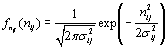(11)

The PDF of residual noiseis: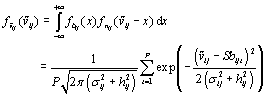(12)

Since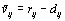, the PDF of r ij becomes: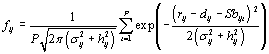(13)

#### CRLB for the case without uncertainty

Substituting (13) intoand, gives: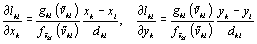(14)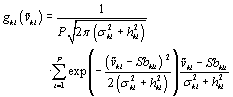(15)

Similarly,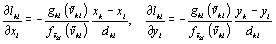(16)

Substituting (14) and (16) into (9), sub-matrices of J include: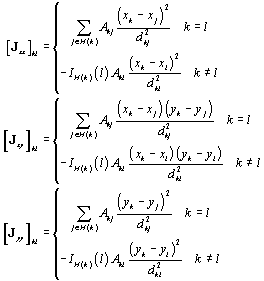(17)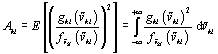(18)

Remark 1 Compared with the FIM in , the FIM of (17) is similar to the FIM in LOS environment. The only difference is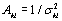when the FIM is derived for LOS environment. Thus, A kl can be rewritten as: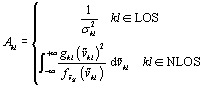(19)

where kl LOS means that the propagation path between the devices k and l is a LOS path, otherwise NLOS path. Equations 17 and 19 give a unified CRLB presentation for both LOS and NLOS environments.

Remark 2 Either empirical model or survey measurements of NLOS errors must be provided since the corresponding CRLB is derived based on the PDF of NLOS errors. Many empirical models and survey measurements of the PDF of NLOS errors are reported in [31, 32].

Remark 3 There are virtually no simple parametric models for the PDF of NLOS errors in all channel environments because its PDF changes as the channel environment changes. It is impossible to derive a CRLB based on parametric method for practical WSNs location system. Thus, the proposed CRLBs based on survey measurements and non-parametric method are necessary since they are applicable for all distributions of NLOS errors.

A particular case where each propagation channel has the same distribution of NLOS errors is considered here. This case will lead to a more compact expression and deeper understanding of CRLB. In this case,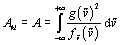(20)

From matrix inversion lemma , the inverse matrix of J is: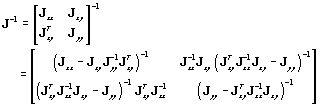(21)

The CRLB can be written as: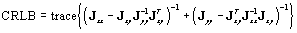(22)

where J xx , J xy , and J yy can be obtained from (17).

From (17), (20), and (22),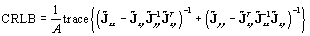(23)

where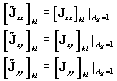(24)

Therefore, the proposed CRLB can be divided into two parts. 1/A in (23) depends on Gaussian noise and NLOS errors while another part consisting of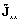,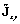, and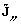is determined by system geometry. For a given geometry, CRLB is proportional to 1/A. Since the CRLBs for LOS and NLOS situations have the same structure, the impacts of geometry on the CRLB in LOS environment can be applicable for the CRLB in NLOS environment. For example, the accuracy increases as more devices are used for location network.

In some special cases, prior information on the locations of BDs may be available for the system. The following CRLB is derived for this case. Assume that the coordinates of BDs θ = [x1...x n y1...y n ]T are subject to a zero-mean Gaussian distribution with covariance matrix Q θ . The PDF of (x i ,y i ), 1 ≤ in can be written as: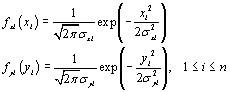(25)

where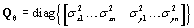.

The log of the joint conditional PDF becomes: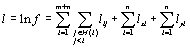(26)

where l ij can be obtained from (7) and (14), l xi = ln f xi (x i ), and l yi = ln f yi (y i ).

The FIM for the case with prior information on the locations of BDs can be obtained by substituting (26) into (5):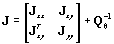where J xx , J xy , and J yy can be obtained from (17).

#### CRLB for the case with uncertainty

The positions of RDs in a practical system provided by GPS receivers may not be exact due to cost and complexity constraints applied on devices. The CRLB considering both NLOS errors and uncertainty of the positions of RDs is needed.

In the presence of disturbances on the positions of RDs, the position of RDs can be modeled as [12, 15]: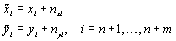(27)

where the disturbances n xi and n yi are assumed to be independent zero-mean Gaussian random variables with varianceand, respectively. The PDF ofand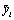can be written as:(28)

The vector of unknown parameters becomes: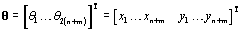(29)

The log of the joint conditional PDF becomes:(30)

Where l ij can be obtained from (7) and (14), l xi = ln f xi (x i ), and l yi = ln f yi (y i ).

Substituting (30) into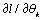, gives: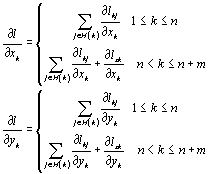(31)

To distinguish from the case without uncertainty, G is used as the FIM. Substituting (31) into (5), the FIM can be rewritten as: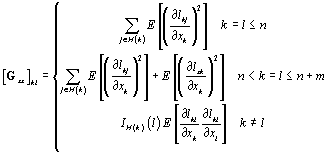(32)

By symmetry, [G yy ] kl have the same structure as [G xx ] kl except that the corresponding x k should be replaced by y k . In addition, [G xy ] kl is the same as [J xy ] kl in (9).

From (28), the derivatives of l xk and l yk are: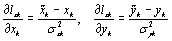(33)

Substituting (14) and (31) into (32), the FIM becomes:(34)

where J xx , J xy , and J yy can be obtained from (17),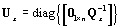,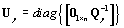,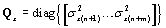, and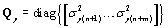.

### Analysis of CRLB

The authors in  proved that the CRLB for the NLOS will become the CRLB for the LOS when NLOS errors go to 0 in CLS and LPS. However, the problem of sensor localization for a WSNs location system becomes more complex since the range measurements among all sensors are used rather than limited measurements between a BD and RDs for a CLS and LPS. Thus, the following Proposition provides theoretical proof for the similar conclusion in WSNs location system. Another purpose of Proposition 1 is to give a robust check for the proposed CRLB.

Proposition 1 In a WSNs location system, the proposed CRLB for NLOS situation will become the CRLB for LOS situation in the case that NLOS errors go to 0.

Proof Consider the case when all the NLOS errors go to 0 (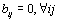) i.e., Sb ijt → 0 and h ij → 0. The limit of PDF (12) is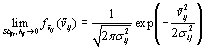(35)

The limit of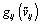is:(36)

Then the limit of A ij can be obtained by substituting (35) and (36) into (18):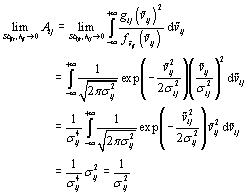(37)

Sinceand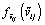are continuous and finite functions, the integral and limit can switch order in (37). Equation 37 also shows that small values of NLOS errors will lead to large A ij . It can be seen from (23) that the CRLB is proportional to 1/A ij . Therefore, large A ij will result in small CRLB. It can be seen from (19) and (37) that the proposed CRLB for NLOS environment reduces to the CRLB derived for LOS environment in  when NLOS errors tend to 0. In other words, the CRLB for LOS environment  can be interpreted as a special case of the proposed CRLB.

### Simulation results

A square region of dimensions 200 m × 200 m is considered for CRLB simulations, where the devices are randomly deployed. The numbers of RDs and BDs are 10 and 50, respectively. The average CRLBs are used to evaluate the performance: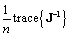(38)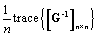(39)

where J and G can be obtained from (17) and (34), respectively. The proposed CRLBs are compared with the CRLB for LOS situation .

#### Case 1: determining the number of samples

The minimum number of samples for achieving relatively accurate results using the derived CRLB is a very important issue. It can be seen from  that non-parametric kernel method can asymptotically converge to any density function with sufficient samples. This implies that the derived CRLBs will converge to their stable values as the number of samples P increases. The minimum P can be determined when the derived CRLBs reach their stable values. In this simulation, NLOS errors are modeled as Rayleigh distribution :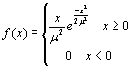(40)

The standard deviation of Gaussian noise is σ ij = 0.1 m and μ is set to 1 which means the mean of NLOS errors is 1.25 m.

Figure 1 shows the derived CRLB versus the number of samples P. It can be observed that the derived CRLB converges to a stable value when P ≥ 230. For the case with insufficient samples, the problem of the determination of the CRLB for WSNs location system in NLOS environments will become unsolvable.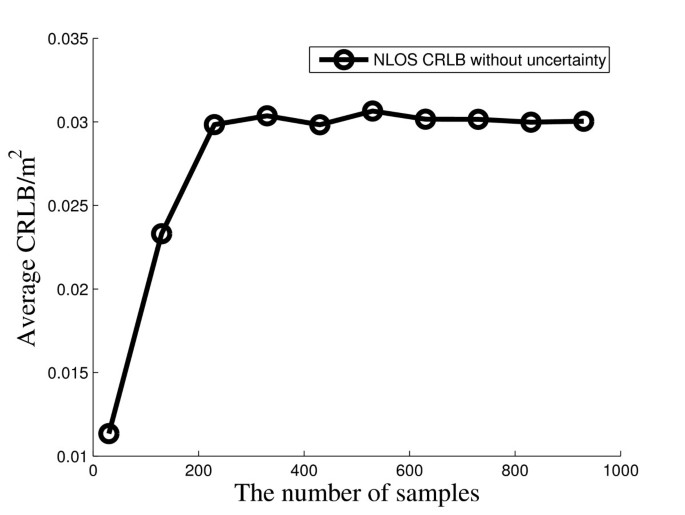Figure 1

#### Case 2: modeling the PDF of NLOS errors by kernel method

This experiment is to evaluate the non-parametric kernel method for estimating the PDF of NLOS errors from survey data. The number of samples can be determined by substituting the survey data of NLOS errors into the derived CRLB and using the method in the above section.

Three different distributions of NLOS errors are considered in this simulation. NLOS errors are first modeled as Rayleigh distribution, and its PDF can be obtained from (40). The theoretical and estimated PDFs of the Rayleigh distribution with μ = 0.1,0.3,1 using the theoretical PDF (40) and estimated PDF (10) are plotted in Figure 2. It can be seen that the theoretical and estimated PDFs are basically the same with different μ.Figure 2

When NLOS errors are modeled as Exponential distribution :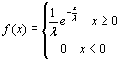(41)

The theoretical and estimated PDFs of the Exponential distribution with λ = 0.1,0.3,1 using the theoretical PDF (41) and estimated PDF (10) are recorded in Figure 3. Figure 3 also shows kernel method can give a good approximation for the PDF of NLOS errors.Figure 3

Compared with parametric estimation method, a major advantage of the kernel method is that it can be used for the PDF without explicit expression. To verify this characteristic, NLOS errors are modeled as: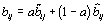(42)

where a is a Bernoulli process with Pr(a = 1) = 0.5. The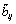andare the Rayleigh and Exponential random variables with μ = 0.3 and λ = 0.3, respectively.

Since the model of NLOS errors described by (42) has no explicit expression for PDF, the frequency histogram and estimated PDF are plotted in Figure 4 by matlab function "hist" and (10), respectively. Figures 2, 3, and 4 show that the proposed equation for PDF estimation (10) is effective.Figure 4

The proposed CRLB is also evaluated in Gaussian noise environment. Since both n ij and b ij are subject to Gaussian distribution, the residual noise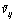is also Gaussian noise. The standard deviation of n ij is σ ij = 0.05 m. Figure 5 shows the CRLBs comparison with different standard deviations of NLOS errors. Compared with the CRLB for LOS environment, the proposed CRLB can provide almost the same bound in LOS environment. The little difference between the two CRLBs may be caused by the randomness of survey data.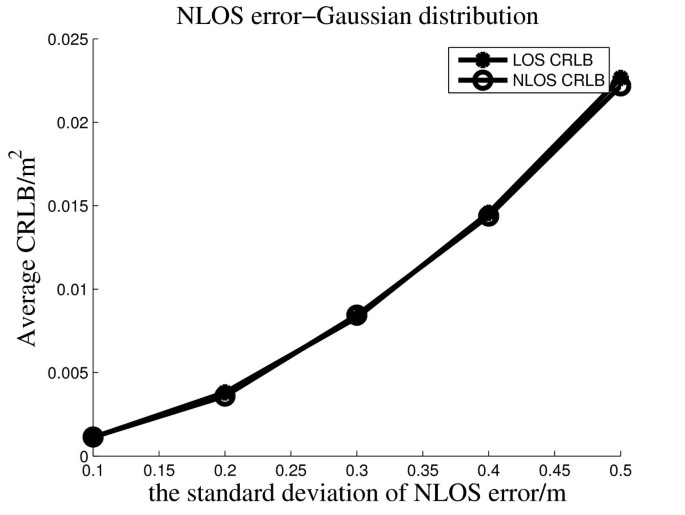Figure 5

#### Case 3: CRLB without uncertainty

Simulations are performed to compare the CRLBs in the case that the positions of RDs are perfectly known. NLOS errors are modeled as the Rayleigh distribution. Figure 6 shows the CRLBs versus the mean of NLOS errors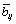with σ ij = 0.1m. It is observed that the proposed CRLB increases as the mean of NLOS errors increases. In all cases, the proposed CRLB is larger than the CRLB for LOS environment. The proposed CRLB will attain the CRLB for LOS environment when NLOS errors become small, which matches Proposition 1.Figure 6

For a practical system it is interesting to study the impacts of the size of the system. Figure 7 shows the CRLBs versus the different sizes of the square region under the conditions that σ ij = 0.1 m and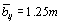. The length of square region is varied from 100 to 400 m with the same geometry. It can be seen that the CRLBs keep the same with different sizes of the system. This means that CRLBs only depend on the noise and geometry whereas the size of the system will not affect the CRLBs since the ratio between the numerator and denominator in (17) has nothing to do with the distance.Figure 7

Figure 8 is performed to study the effects of the number of devices on the CRLBs for NLOS environment. The numbers of BDs n and RDs m are varied from 10 to 100 and 4 to 20, respectively. Let σ ij = 0.1 m and. Figure 8 shows that the CRLB decreases as n and m increase. Although both of n and m can help to improve the positioning accuracy, the number of RDs is more useful than that of BDs as shown in Figure 8. However, increasing m will lead to more costs. It is necessary to find a balance between the system performance and costs according to the practical requirement.Figure 8

#### Case 4: CRLB with uncertainty

The differences between the two proposed CRLBs are considered here. One is derived for the case with uncertainty (34) and another is for the case without uncertainty (17). The two CRLBs versus the mean of NLOS errorswith σ ij = 0.1 and σ xi = σ yi = 0.2 are recorded in Figure 9. Figure 9 shows that both CRLBs increase as the mean of NLOS errors increases. Figure 10 shows the two proposed CRLBs versus the standard deviation (SD) of the errors in the positions of RDs. It is shown that the positioning accuracy decreases as the standard deviation increases. Figures 9 and 10 show that even little error of RD position will greatly reduce the positioning accuracy. It can also be seen that the case without uncertainty has the lower CRLB than the case with uncertainty.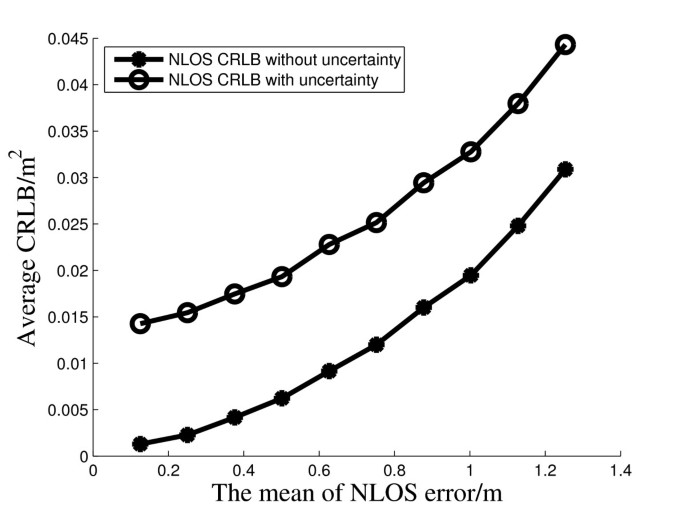Figure 9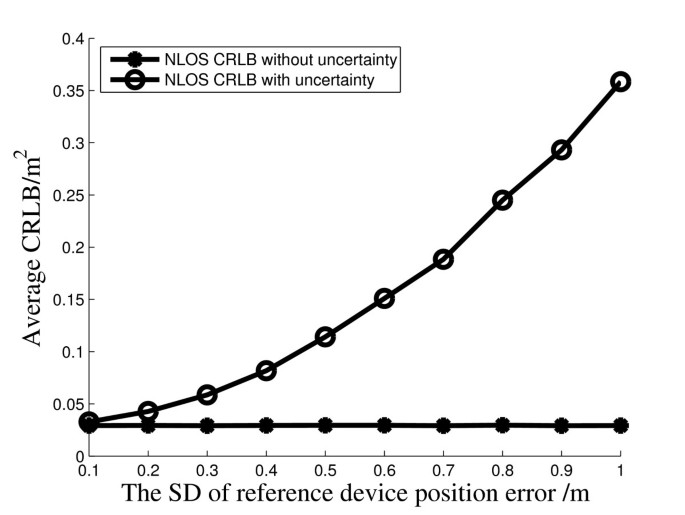Figure 10

## Conclusions

The performance of WSNs location system in NLOS environment is analyzed in this article. The best positioning accuracy is evaluated in terms of the CRLBs. Since non-parametric kernel method is used to build the PDF of NLOS errors, the proposed CRLBs are suitable for various distributions in different channel environments. The proposed CRLBs consider both the cases that the positions of RDs are perfectly or imperfectly known. In addition, the relationship between the CRLBs for LOS and NLOS environments is given. The article shows that the CRLB for LOS environment  can be interpreted as a special case of the proposed CRLB, when NLOS errors go to 0.

## Abbreviations

BDs:

blindfolded devices

CRLB:

Cramer-Rao lower bound

FIM:

Fisher information matrix

GPS:

global positioning system

LOS:

line-of-sight

MDS:

multi-dimensional scaling

NLOS:

non-line-of-sight

RDs:

reference devices

SD:

standard deviation

TOA:

time-of-arrival

WSNs:

wireless sensor networks.

## References

1. 1.

Akyildiz IF, Su W, Sankarasubramaniam Y, Cayirci E: A survey on sensor networks. IEEE Commun Mag 2002, 40(8):102-114. 10.1109/MCOM.2002.1024422

2. 2.

Patwari N, Ash JN, Kyperountas S, Hero AO III, Moses RL, Correal NS: Locating the nodes: cooperative localization in wireless sensor networks, IEEE Signal Process. Mag 2005, 22(4):54-69.

3. 3.

Wang H, Elson J, Girod L, Estrin D, Yao K: Target classification and localization in habitat monitoring. IEEE International Conference on Acoustics, Speech, and Signal Processing 2003, 4: 6-10.

4. 4.

Ji X, Zha HY: Sensor positioning in wireless ad-hoc sensor networks using multidimensional scaling. Twenty-third Annual Joint Conference of the IEEE Computer and Communications Societies 2004, 7: 2652-2661.

5. 5.

Latsoudas G, Sidiropoulos ND: A two-stage fastmap-MDS approach for node localization in sensor networks. 2005 1st IEEE International Workshop on Computational Advances in Multi-Sensor Adaptive Processing 2005, 64-67.

6. 6.

Ahmed AA, Shang Y, Shi HC: Variants of multidimensional scaling for node localization. 11th International Conference on Parallel and Distributed Systems 2005, 1: 140-146.

7. 7.

Wu CH, Sheng WH, Zhang Y: Mobile sensor networks self localization based on multi-dimensional scaling. 2007 IEEE International Conference on Robotics and Automation 2007, 10-14.

8. 8.

Birchfield ST: Geometric microphone array calibration by multidimensional scaling. IEEE International Conference on Acoustics, Speech, and Signal Processing 2003, 5: 57-60.

9. 9.

Costa JA, Patwari N, Hero AO: Achieving high-accuracy distributed localization in sensor networks. IEEE International Conference on Acoustics, Speech, and Signal Processing 2005, 3: 18-23.

10. 10.

Biaz S, Ji YM: Precise distributed localization algorithms for wireless networks. Sixth IEEE International Symposium on World of Wireless Mobile and Multimedia Networks 2005, 388-394.

11. 11.

Vo N, Vo D, Lee SY, Challa S: Weighted nonmetric MDS for sensor localization. International Conference on Advanced Technologies for Communications 2008, 53: 391-394.

12. 12.

Lui KWK, Ma WK, So HC, Chan FKW: Semi-definite programming algorithms for sensor network node localization with uncertainties in anchor positions and/or propagation speed. IEEE Trans Signal Processing 2009, 57(2):752-763.

13. 13.

Patwari N, Hero AO III, Perkins M, Correal NS, O'Dea RJ: Relative location estimation in wireless sensor networks. IEEE Trans Signal Process 2003, 51(8):2137-2148. 10.1109/TSP.2003.814469

14. 14.

Jia T, Buehrer RM: A new Cramer-Rao lower bound for TOA-based localization. Military Communications Conference 2008, 1-5.

15. 15.

Yu K, Guo YJ: Anchor Global Position Accuracy Enhancement Based on Data Fusion. IEEE Trans Veh Technol 2008, 58(3):1616-1623.

16. 16.

Larsson EG: Cramer-Rao bound analysis of distributed positioning in sensor networks. IEEE Signal Process Lett 2004, 11(3):334-337. 10.1109/LSP.2003.822899

17. 17.

Chang C, Sahai A: Cramer-Rao-type bounds for localization. EURASIP J Appl Signal Process 2006, 58(7):1-13.

18. 18.

Chen P-C: A non-line-of-sight error mitigation algorithm in location estimation. Proceedings of the IEEE WCNC 1999, 316-320.

19. 19.

Kim W, Lee JG, Jee G-I: The interior-point method for an optimal treatment of bias in trilateration location. IEEE Trans Veh Technol 2006, 55(4):1291-1301. 10.1109/TVT.2006.877760

20. 20.

Feng KT, Chen CL, Chen CH: GALE: an enhanced geometry-assisted location estimation algorithm for NLOS environments. IEEE Trans Mobile Comput 2008, 7(2):199-213.

21. 21.

Miao H, Yu K, Juntti M: Positioning for NLOS propagation: Algorithm derivations and Cramer-Rao bounds. IEEE Trans Veh Technol 2007, 56(5):2568-2580.

22. 22.

Qi Y, Kobayashi H, Suda H: Analysis of wireless geolocation in a non-line-of-sight environment. IEEE Trans Wireless Commun 2006, 5(3):672-681.

23. 23.

Yuan S, Win MZ: Fundamental limits of wideband localization--part I: a general framework. IEEE Trans Inf Theory 2010, 56(10):4956-4980.

24. 24.

Yuan S, Wymeersch H, Win MZ: Fundamental limits of wideband localization--part ii: cooperative networks. IEEE Trans Inf Theory 2010, 56(10):4981-5000.

25. 25.

Jourdan D, Dardari D, Win M: Position error bound for UWB localization in dense cluttered environments. IEEE Trans Aerospace Electron Syst 2008, 44(2):613-628.

26. 26.

Mazuelas S, Lorenzo RM, Bahillo A, Fernandez P, Prieto J, Abril EJ: Topology assessment provided by weighted barycentric parameters in harsh environment wireless location systems. IEEE Trans Signal Process 2010, 58(7):3842-3857.

27. 27.

Kay SM: Fundamentals of Statistical Signal Processing: Estimation Theory. Prentice-Hall, Englewood Cliffs; 1993.

28. 28.

Van HL: Detection. In Estimation, and Modulation Theory, Part I: Detection, Estimation, and Linear Modulation Theory. Wiley, New York; 2001.

29. 29.

Mcguire M, Platuniotis KN, Venetsanopoulos AN: Location of mobile terminals using time measurements and survey points. IEEE Trans Veh Technol 2003, 52(4):999-1011. 10.1109/TVT.2003.814222

30. 30.

Elgammal A, Duraiswami R, Harwood D, Davis LS: Background and foreground modeling using nonparametric kernel density estimation for visual surveillance. Proc IEEE 2002, 90(7):1151-1163. 10.1109/JPROC.2002.801448

31. 31.

Destino G, Macagnano D, de Abreu GTF: Hypothesis testing and iterative WLS minimization for WSN localization under LOS/NLOS conditions. The Forty-First Asilomar Conference on Signals, Systems and Computers 2007, 2150-2155.

32. 32.

Prieto J, Bahillo A, Mazuelas S, Lorenzo RM, Blas J, Fernandez P: NLOS mitigation based on range estimation error characterization in an RTT-based IEEE 802.11, indoor location system. IEEE International Symposium on Intelligent Signal Processing 2009, 61-66.

33. 33.

Horn RA, Johnson CR: Matrix Analysis. Cambridge University Press, Cambridge; 1999.

34. 34.

Elgammal A, Duraiswami R, Harwood D, Davis LS: Background and foreground modeling using nonparametric kernel density estimation for visual surveillance. Proc IEEE 2002, 90(7):1151-1163. 10.1109/JPROC.2002.801448

Download references

## Acknowledgements

This study is sponsored by the Intelligent Energy Distribution Systems Thematic Research Grant from A*STAR Science and Engineering Research Council, Singapore and supported by the Youth Fund of the University of Electronic Science and Technology of China (L08010201JX0826), and the Fundamental Research Funds for the Central Universities (ZYGX2009J018).

## Author information

Authors

### Corresponding author

Correspondence to Jiyan Huang.

## Additional information

### Competing interests

The authors declare that they have no competing interests.

## Authors’ original submitted files for images

Below are the links to the authors’ original submitted files for images.

## Rights and permissions

Open Access This article is distributed under the terms of the Creative Commons Attribution 2.0 International License (https://creativecommons.org/licenses/by/2.0), which permits unrestricted use, distribution, and reproduction in any medium, provided the original work is properly cited.

Reprints and Permissions

## About this article

### Cite this article

Huang, J., Wang, P. & Wan, Q. CRLBs for WSNs localization in NLOS environment. J Wireless Com Network 2011, 16 (2011). https://doi.org/10.1186/1687-1499-2011-16

Download citation

• Received:

• Accepted:

• Published:

### Keywords

• Wireless sensor networks
• Cramer-Rao lower bound
• Non-line-of-sight
• Node localization